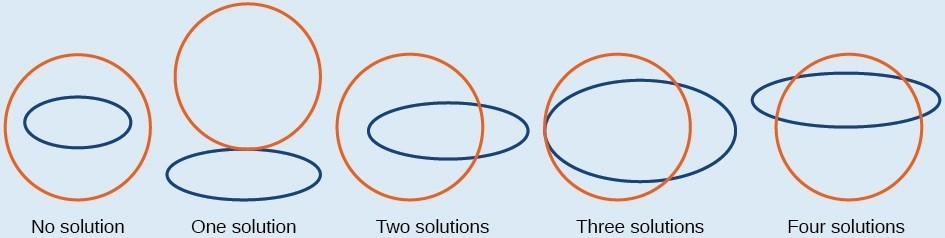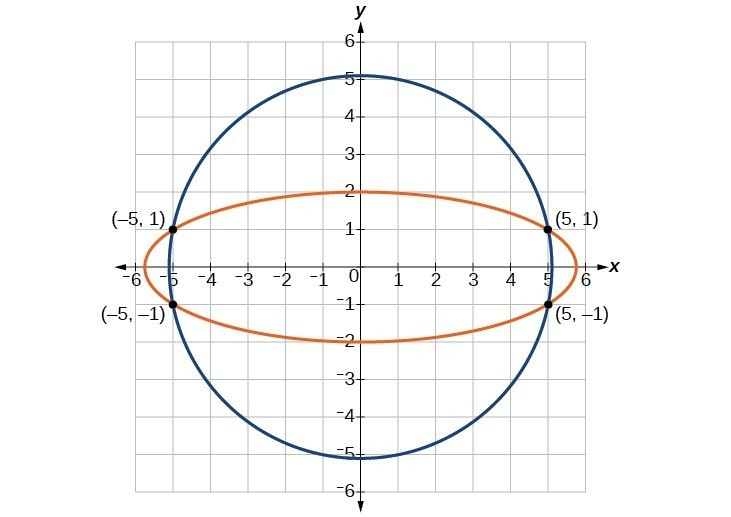## Solving a System of Nonlinear Equations Using Elimination

We have seen that substitution is often the preferred method when a system of equations includes a linear equation and a nonlinear equation. However, when both equations in the system have like variables of the second degree, solving them using elimination by addition is often easier than substitution. Generally, elimination is a far simpler method when the system involves only two equations in two variables (a two-by-two system), rather than a three-by-three system, as there are fewer steps. As an example, we will investigate the possible types of solutions when solving a system of equations representing a circle and an ellipse.

### A General Note: Possible Types of Solutions for the Points of Intersection of a Circle and an Ellipse

Figure 6 illustrates possible solution sets for a system of equations involving a circle and an ellipse.

• No solution. The circle and ellipse do not intersect. One shape is inside the other or the circle and the ellipse are a distance away from the other.
• One solution. The circle and ellipse are tangent to each other, and intersect at exactly one point.
• Two solutions. The circle and the ellipse intersect at two points.
• Three solutions. The circle and the ellipse intersect at three points.
• Four solutions. The circle and the ellipse intersect at four points.Figure 6

### Example 3: Solving a System of Nonlinear Equations Representing a Circle and an Ellipse

Solve the system of nonlinear equations.

$\begin{array}{rr}\hfill {x}^{2}+{y}^{2}=26& \hfill \left(1\right)\\ \hfill 3{x}^{2}+25{y}^{2}=100& \hfill \left(2\right)\end{array}$

### Solution

Let’s begin by multiplying equation (1) by $-3,\text{}$ and adding it to equation (2).

$\frac{\begin{array}{l}\begin{array}{l}\hfill \\ \left(-3\right)\left({x}^{2}+{y}^{2}\right)=\left(-3\right)\left(26\right)\hfill \\ \text{ }-3{x}^{2}-3{y}^{2}=-78\hfill \end{array}\hfill \\ \text{ }3{x}^{2}+25{y}^{2}=100\hfill \end{array}}{\text{ }22{y}^{2}=22}$

After we add the two equations together, we solve for $y$.

$\begin{array}{l}{y}^{2}=1\hfill \\ y=\pm \sqrt{1}=\pm 1\hfill \end{array}$

Substitute $y=\pm 1$ into one of the equations and solve for $x$.

$\begin{array}{l}\text{ }{x}^{2}+{\left(1\right)}^{2}=26\hfill \\ \text{ }{x}^{2}+1=26\hfill \\ \text{ }{x}^{2}=25\hfill \\ \text{ }x=\pm \sqrt{25}=\pm 5\hfill \\ \hfill \\ {x}^{2}+{\left(-1\right)}^{2}=26\hfill \\ \text{ }{x}^{2}+1=26\hfill \\ \text{ }{x}^{2}=25=\pm 5\hfill \end{array}$

There are four solutions: $\left(5,1\right),\left(-5,1\right),\left(5,-1\right),\text{and}\left(-5,-1\right)$.Figure 7

### Try It 3

Find the solution set for the given system of nonlinear equations.

$\begin{array}{c}4{x}^{2}+{y}^{2}=13\\ {x}^{2}+{y}^{2}=10\end{array}$

Solution